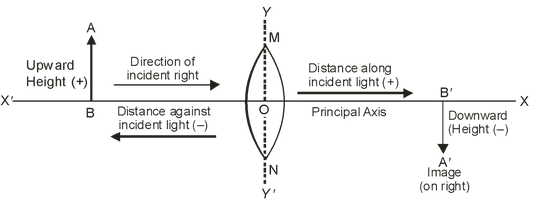# Sign Convection For Refraction By Spherical Lenses

## Light of Class 10

### SIGN CONVENTION FOR REFRACTION BY SPHERICAL LENSES

The New Cartesian Sign Convention is used for measuring the various distances in the ray-diagrams of spherical lenses (convex as well as concave spherical lenses).

According to the New Cartesian Convention.

•  The optical centre ‘O’ of the lens is taken as the origin and the principal axis of the lens is taken as the x-axis of the co-ordinate system.
• The object is always placed to the left of the lens i.e. the light (incident rays) from the object falls on the lens from the left hand side.
•  All the distances parallel to the principal axis of the spherical lenses are measured from the optical centre ‘O’ of the lenses.
•  All the distances measured to the right of the origin (along +ve x-axis) are taken as positive.
•  All the distances measured to the left of the origin (along –ve x-axis) are taken as negative.
•  The distances (heights) measured upwards (i.e. above the x-axis) and perpendicular to the principal axis of the lens are taken as positive.
• The distances (heights) measured downwards (i.e. below the x-axis) and perpendicular to the principal axis of the lens are taken as negative. The following figure illustrates all the points of the New Cartesian. Sign Convention stated above